# Excel VBA Logical Operators

• Last Updated : 24 Aug, 2021

Logical operators are used for performing logical and asthmatic operations on a set of values or variables. The table depicts all the different types of logical operators supported by Excel:

### 1. AND  (LOGICAL AND)

If both the conditions are True, then the Expression is true.

Example:

Assume variable A holds 20 and variable B holds 0, then a<>0 AND b<>0 is False

Program:

```Private Sub Demo_Loop()

Dim a As Integer //Declaring variable

a = 20

Dim b As Integer  //Declaring variable

b = 0

If a <> 0 And b <> 0 Then

MsgBox ("AND LOGICAL Operator Result is : True")

Else

MsgBox ("AND LOGICAL Operator Result is : False")

End If

End Sub```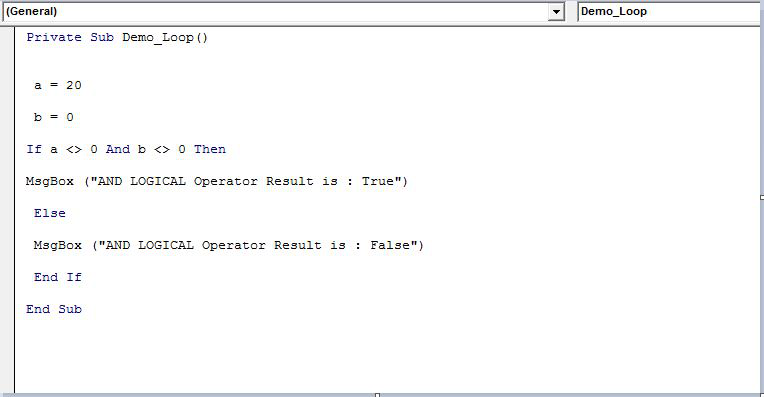Output:

`AND LOGICAL Operator Result is : False`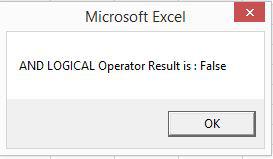### 2. OR( Logical OR Operator)

If any of the two conditions are True, then the condition is true.

Example:

Assume variable A holds 20 and variable B holds 0, then a<>0 OR b<>0 is true.

Program:

```Private Sub Demo_Loop()
Dim a As Integer //Declaring variable
a = 20
Dim b As Integer  //Declaring variable
b = 0
If a <> 0 Or b <> 0 Then
MsgBox ("OR LOGICAL Operator Result is : True")
Else
MsgBox ("OR LOGICAL Operator Result is : False")
End If
End Sub```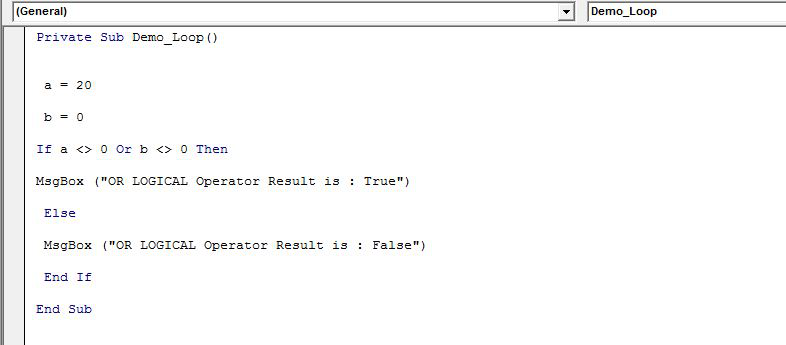Output:

`OR LOGICAL Operator Result is : True`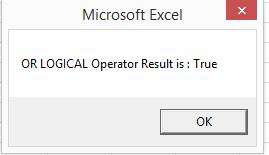### 3. NOT( Logical NOT Operator)

Reverse the result. If a condition is true, then the Logical NOT operator will make false.

Example:

Assume variable A holds 20 and variable B holds 0, then NOT(a<>0 OR b<>0) is false.

Program:

```Private Sub Demo_Loop()
Dim a As Integer //Declaring variable
a = 20
Dim b As Integer  //Declaring variable
b = 0
If a <> 0 Not b <> 0 Then
MsgBox ("NOT LOGICAL Operator Result is : True")
Else
MsgBox ("NOT LOGICAL Operator Result is : False")
End If
End Sub```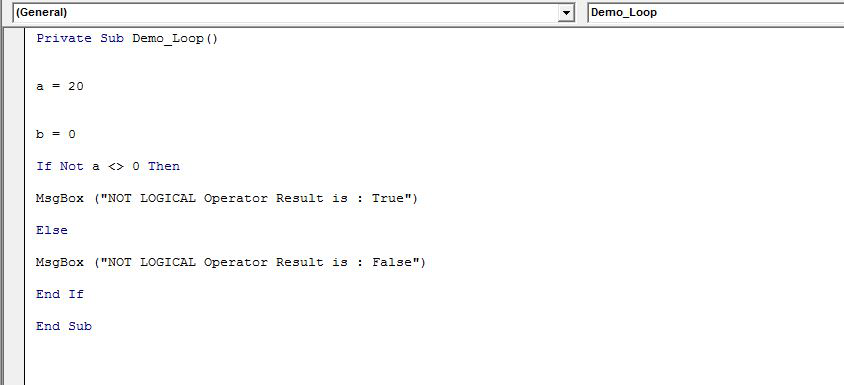Output:

`NOT LOGICAL Operator Result is : False`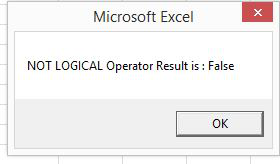### 4. XOR( Logical XOR Operator)

It is the combination of NOT and OR Operator. If one, and only one, of the expressions, evaluate to be True, the result is True.

Example:

Assume variable A holds 20 and variable B holds 0, then (a<>0 XOR b<>0) is true.

Program:

```Private Sub Demo_Loop()
Dim a As Integer //Declaring variable
a = 20
Dim b As Integer  //Declaring variable
b = 0
If a <> 0 Xor b <> 0 Then
MsgBox ("XOR LOGICAL Operator Result is : True")
Else
MsgBox ("XOR LOGICAL Operator Result is : False")
End If
End Sub```Output:

`XOR LOGICAL Operator Result is : True`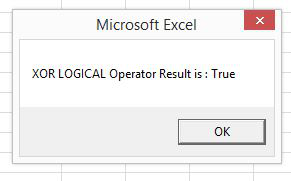A Sample Program showing all the Operators is included below along with the outputs: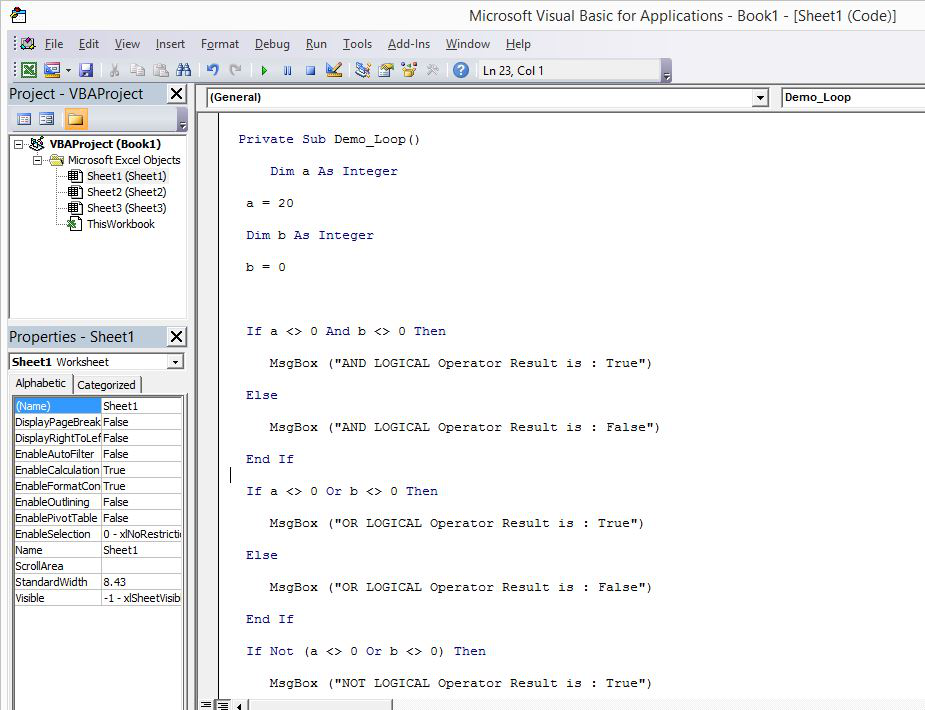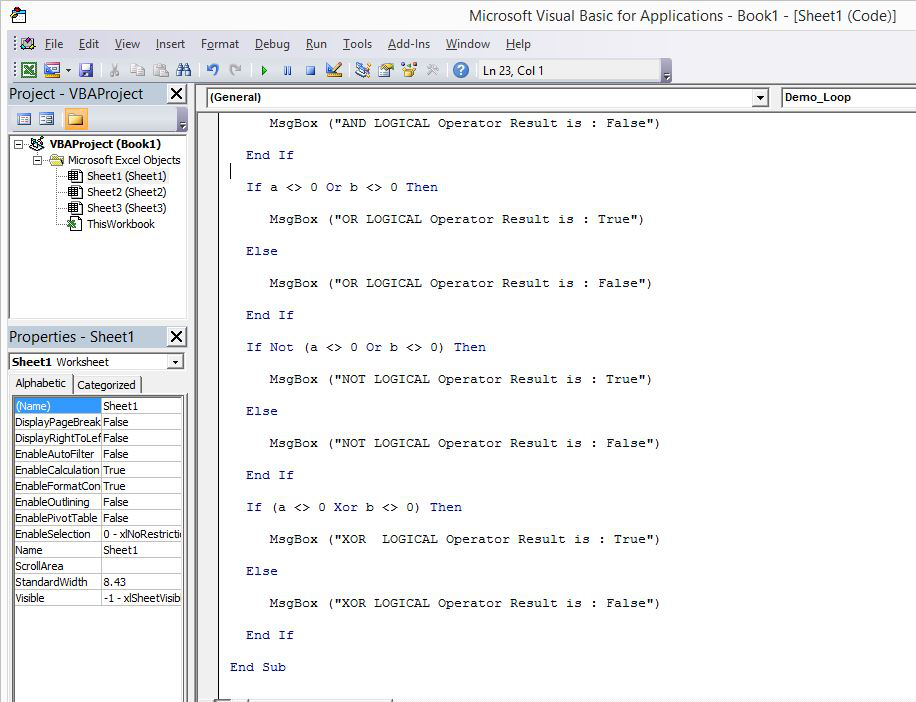Program:

``` Private Sub Demo_Loop()
Dim a As Integer //Declaring variable
a = 20
Dim b As Integer  //Declaring variable
b = 0

If a <> 0 And b <> 0 Then
MsgBox ("AND LOGICAL Operator Result is : True")
Else
MsgBox ("AND LOGICAL Operator Result is : False")
End If
If a <> 0 Or b <> 0 Then
MsgBox ("OR LOGICAL Operator Result is : True")
Else
MsgBox ("OR LOGICAL Operator Result is : False")
End If
If Not (a <> 0 Or b <> 0) Then
MsgBox ("NOT LOGICAL Operator Result is : True")
Else
MsgBox ("NOT LOGICAL Operator Result is : False")
End If
If (a <> 0 Xor b <> 0) Then
MsgBox ("XOR  LOGICAL Operator Result is : True")
Else
MsgBox ("XOR LOGICAL Operator Result is : False")
End If
End Sub```

Output:

```AND LOGICAL Operator Result is : False
OR LOGICAL Operator Result is : True
NOT LOGICAL Operator Result is : False
XOR LOGICAL Operator Result is : True```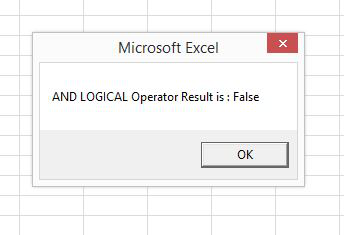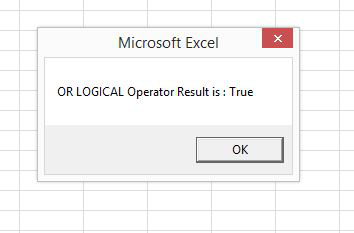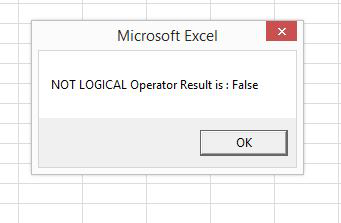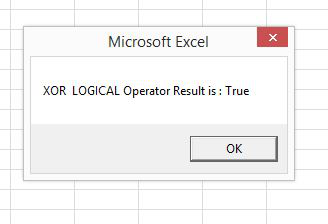My Personal Notes arrow_drop_up